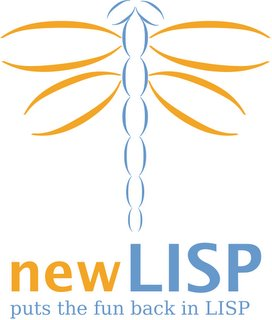### Eval and Anti-eval.;---------------------------------------------------------------
; I again need some functions and macros I already defined.
; For the rest of the text see bellow.

(set 'macro
(lambda-macro()
(eval (append '(lambda-macro) (args)))))

(set 'expression-and-value
(macro(expression-and-value-arg)
(list '->
expression-and-value-arg
(eval expression-and-value-arg))))

(set '§ (macro()
(doargs(§arg)
(println (eval (list 'expression-and-value §arg))))))

;---------------------------------------------------------------
;
;                  Eval and Anti-eval.
;
; Quote and eval can be seen as inverse functions;
; But, they are not exactly that:

(set 'x "I am the value of x")

(println (= x (quote (eval x))))  ; -> nil! UGLY! UGLY!
(println (= x (eval (quote x))))  ; -> true

; What really happens?

(§ (quote (eval x)))  ;(-> (quote (eval x)) (eval x))
(§ (eval (quote x)))  ;(-> (eval (quote x)) "I am the value of x")

; Say we want real inverse of eval, i.e. function anti-eval
; such that everything works as expected.

(set 'anti-eval
(macro(argument)
(if (and (list? argument)
(not (empty? argument))
(= (first argument) 'eval))
(eval (first (rest argument)))
argument)))

;Tests:

(§ (anti-eval (eval x)))
(§ (eval (anti-eval x)))

(§ (anti-eval (eval (quote (+ 2 3)))))
(§ (eval (anti-eval (quote (+ 2 3)))))

(§ (eval (anti-eval (eval (anti-eval x)))))
(§ (anti-eval (eval (anti-eval (eval x)))))

;Results:

;(-> (anti-eval (eval x)) "I am the value of x")
;(-> (eval (anti-eval x)) "I am the value of x")

;(-> (anti-eval (eval (quote (+ 2 3)))) (+ 2 3))
;(-> (eval (anti-eval (quote (+ 2 3)))) (+ 2 3))

;(-> (eval (anti-eval (eval (anti-eval x)))) "I am the value of x")
;(-> (anti-eval (eval (anti-eval (eval x)))) "I am the value of x")

;So far, so good.

;And even this one:

(§ (eval (anti-eval (anti-eval (eval x)))))
(§ (anti-eval (eval (eval (anti-eval x)))))

;(-> (eval (anti-eval (anti-eval (eval x)))) "I am the value of x")
;(-> (anti-eval (eval (eval (anti-eval x)))) "I am the value of x")

; But I wouldn't be surprised it is broken for more complicated
; expression. I'll keep an eye on it. But this is only blog.

; Is there any practical use of anti-eval? I don't know,
; but it would be strange that there is no use whatsoever.

#### 3 comments:

1.Good post! These are very interesting ideas.

One question: Why do you say this:

(println (= x (quote (eval x)))) ; -> nil! UGLY! UGLY!

Why is this really ugly to you? To me (unburdened by knowledge of Common Lisp or Scheme), it looks like asking whether an evaluated symbol is equal to an unevaluated entity.

2.You are right, it has a perfect sense in Lisp and Newlisp.

It is ugly only because some inverse functions in mathematics behave on prettier way, i.e. exp and log, differentiation and integration. It is simpler to think about these pairs.

So, I was provoked by the question, is it possible to have something that is full left and right side inverse to eval.

And can it be useful?

Actually, answer on that last question is already known - it can, that is why CL and Scheme provide something similar, i.e

(= 3 (quasiquote (unquote 3))) ;=> true

i.e. quasiquote behave just as quote - except unquote - which is something similar to eval - is able to "fight against" quasiquote not from outside (like eval fight against quote) but from inside.

But, again, unquote cannot fight against quasiquote from outside.

(= 3 (unquote (quasiquote 3)) => ERROR.

However, eval can do that.

(= 3 (eval (quasiquote 3))) => true

I somehow think it also turned to be too complicated and there should be some generalized concept that on a mathematically elegant way covers all of the functionalities of quote, eval, quasiquote and unquote - and maybe beyond.

I just play a bit in that direction with this blog entry.

3.These examples of quasiquote are from Scheme, it is similar (but I'd say worse in CL) where ` is used instead of quasiquote and , instead of unquote. Surprisingly, in CL

(equal ``3 ''3) => T